WOW !! MUCH LOVE ! SO WORLD PEACE !
Fond bitcoin pour l'amélioration du site: 1memzGeKS7CB3ECNkzSn2qHwxU6NZoJ8o
Dogecoin (tips/pourboires): DCLoo9Dd4qECqpMLurdgGnaoqbftj16NvpRechercher sur le site:

# Health risk assessment associated with the reuse of compost, urine and greywater in agricultural field in sahelian climate.

( Télécharger le fichier original )
par Alexis Loukou BROU
Fondation 2iE - Master Environnement option Eau et Assainissement 2014

### 3.2.2. From consumers

According to Shuval et al., (1997) 10.8 mL of irrigation water will be left on a 100 g lettuce after harvest. There are two days between lettuce harvest and consumption (WHO, 2006a). The amount of lettuce consumed per person per day was taken as 100 g at a rate of one lettuce per week per consumerin developing country (Shuval et al., 1997);(Nana O.B. Ackerson and Esi Awuah, 2012)such as Burkina Faso. Thus, a consumer can be exposed 52 times per year.The exposure scenarios of different matrix for farmers and consumers are summarized in table 3 below.

Table 3: Different exposure scenarios and pathways which farmers and consumers can be exposed in different cases

 Target population Matrix of manipulation Exposure scenario Quantityingested Frequencyexposed (events/year) Farmers Compost Handle without protection individual (glove, mask,...) before to spread compost 10-100mga 5 Urine Handle urine in the field with a small bucket and use this hand to eat without washing it 0.43 mL* 15 Soil Ingestion of soil contaminated with greywater, compost orurine. 10-100mgb 20 Greywater Ingestion of greywater from the irrigation system (watering cans or bucket ) Accidental ingestion 1-2mLc 275 Consumers Lettuceharvest Consumers can eat lettuce without washing it 10.8mL/100gd 52

a=(Schönning et al., 2007) ; b=(Haas et al., 1999) ; c=(Nana O.B. Ackerson and Esi Awuah, 2012); d=(Shuval et al., 1997).*= Protocol of determination of amount of urine ingested (annex 2).

### 3.3. Dose-response assessment

For dose-response relationships, the beta-Poisson dose-response model described by Haas et al., (1999)was used for Salmonella, Ascaris. However, single-hit exponential dose-response can be applied for Salmonella and Ascaris. Dose-response parameters for exponential and beta-Poisson models from various enteric pathogen ingestion studied by different authors were summarized in table 4 below. To calculate microbial risk, uncertain values (minimum and maximum values) of pathogen amounts will use to evaluate risk for each treatment.

Single-hit exponential model: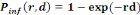(Equation 5)

Beta-Poisson model: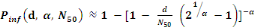(Equation 6)

Where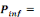the probability of infection which is a function of r and d= empirical parameter assumed to be constant for any given host and given pathogen picked to fit the data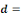Mean ingested dose, N50= the median dose, á andâ= slope parameters, which hold when â=1 and á=â.

The annual probability of infection is given by: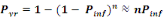(Equation 7)

Where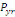= acceptable annual risk of infection caused by a pathogenic organism

n = number of exposure events per year (events/yr).

A QMRA model for broccoli, cucumber, lettuce, and three cultivars of cabbage constructed by Hamilton et al. (2006) was used to calculate the daily dose of pathogenic organism on the lettuce. The beta -Poisson and exponential dose -response models were subsequently used to calculate the probability of infection (Nana O.B. Ackerson and Esi Awuah, 2012).

The daily dose of pathogens, ë=d, taken as a result of consuming the lettuce was calculated as: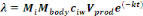(Equation 8)

Where,

Mbody = human body mass (kg)

Mi = daily consumption per capita per kg of body mass [g (kg.ca.day)-1]

ciw= concentration of pathogens in irrigation water

Vprod= volume of irrigation water caught by product (mL.g-1)

k = pathogen kinetic decay constant (day-1)

t = time between last reclaimed - water irrigation event and harvest/consumption/storage (day).

Mbody = 71.8 kg

From survey, Mi = 1.6713 g. (kg.ca.day)-1

Vprod = 0.125 mL g-1 ; t = 2 d.

Table 4: Summary of dose-response parameters for exponential and beta-Poisson models from various enteric pathogen ingestion studies

 ` ` Exponential beta-Poisson Constituent r á â N50 Escherichia coli ` ` 0.1705a 1.61 x 106a ` ` Salmonella 0,00752a 0,313b ` ` 23600b Ascaris 1b 0,104c ` ` 859c

a= (Metcalf & Eddy, 2007); b= (Schönning et al., 2007); c= (Mara and Sleigh, 2010b)

BOSKELYWOOD from Ona Luambo on Vimeo.